Samacheer Kalvi 12th Physics Solutions Chapter 1 Electrostatics - ACRP.in

# Samacheer Kalvi 12th Physics Solutions Chapter 1 Electrostatics

## Tamilnadu Samacheer Kalvi 12th Physics Solutions Chapter 1 Electrostatics

### Samacheer Kalvi 12th Physics Electrostatics Textual Evaluation Solved

Samacheer Kalvi 12th Physics Electrostatics Multiple Choice Questions

Question 1.
Two identical point charges of magnitude -q are fixed as shown in the figure below. A third charge +q is placed midway between the two charges at the point P. Suppose this charge +q is displaced a small distance from the point P in the directions indicated by the arrows, in which direction(s) will +q be stable with respect to the displacement?

(a) A1 and A2
(b) B1 and B2
(c) both directions
(d) No stable
(b) B1 and B2

Question 2.
Which charge configuration produces a uniform electric field?
(a) point charge
(b) the infinite uniform line charge
(c) uniformly charged infinite plane
(d) uniformly charged spherical shell
(c) uniformly charged infinite plane

Question 3.
What is the ratio of the charges ∣∣q1q2∣∣ for the following electric field line pattern?

(a) 15
(b) 2511
(c) 5
(d) 1225
(d) 1225

Question 4.
An electric dipole is placed at an alignment angle of 30° with an electric field of 2 x 105 N C-1. It experiences a torque equal to 8 N m. The charge on the dipole if the dipole length is 1 cm is-
(a) 4 mC
(b) 8 mC
(c) 5 mC
(d) 1 mC
(b) 8 mC

Question 5.
Four Gaussian surfaces are given below with charges inside each Gaussian surface. Rank the electric flux through each Gaussian surface in increasing order-

(a) D < C < B < A
(b) A < B = C < D
(c) C < A = B < D
(d)D > C > B > A
(a) D < C < B < A

Question 6.
The total electric flux for the following closed surface which is kept inside water-

(a) 80qε0
(b) q40ε0
(c) q80ε0
(d) q40ε0
(b) q40ε0

Question 7.
Two identical conducting balls having positive charges q1 and q2 are separated by a center to center distance r. If they are made to touch each other and then separated to the same distance, the force between them will be- (NSEP 04-05)
(a) less than before
(b) same as before
(c) more than before
(d) zero
(c) more than before

Question 8.
Rank the electrostatic potential energies for the given system of charges in increasing order

(a) 1 = 4 < 2 < 3
(b) 2 = 4 < 3 < 1
(c) 2 = 3 < 1 < 4
(d) 3 < 1 < 2 < 4
(a) 1 = 4 < 2 < 3

Question 9.
An electric field E⃗  = 10xi^ exists in a certain region of space. Then the potential difference V = V0 – VA, Where V0 is the potential at the origin and VA is the potential at x = 2 m is-
(a) 10 J
(b) -20 J
(c) + 20 J
(d) – 10 J
(a) 10 J

Question 10.
A thin conducting spherical shell of radius R has a charge Q which is uniformly distributed on its surface. The correct plot for electrostatic potential due to this spherical shell is-

Question 11.
Two points A and B are maintained at a potential of 7 V and -4 V respectively. The work done in moving 50 electrons from A to B is-
(a) 8.80 x 10-17 J
(b) -8.80 x 10-17 J
(c) 4.40 x 10-17 J
(d) 5.80 x 10-17 J
(a) 8.80 x 10-17 J

Question 12.
If voltage applied on a capacitor is increased from V to 2V, choose the correct conclusion.
(a) Q remains the same, C is doubled
(b) Q is doubled, C doubled
(c) C remains same, Q doubled
(d) Both Q and C remain same
(c) C remains same, Q doubled

Question 13.
A parallel plate capacitor stores a charge Q at a voltage V. Suppose the area of the parallel plate capacitor and the distance between the plates are each doubled then which is the quantity that will change?
(a) Capacitance
(b) Charge
(c) Voltage
(d) Energy density
(d) Energy density

Question 14.
Three capacitors are connected in a triangle as shown in the figure. The equivalent capacitance between points A and C is

(a) 1 μF
(b) 2 μF
(c) 3 μF
(d) 14 μF
(b) 2 μF

Question 15.
Two metallic spheres of radii 1 cm and 3 cm are given charges of -1 x 10-2 C and 5 x 10-2 C respectively. If these are connected by a conducting wire, the final charge on the bigger sphere is (AIIPMT 2012)
(a) 3 x 10-2 C
(b) 4 x 10-2 C
(c) 1 x 10-2 C
(d) 2 x 10-2 C
(a) 3 x 10-2 C

Samacheer Kalvi 12th Physics Electrostatics Short Answer Questions

Question 1.
What is meant by quantisation of charges?
The charge q on any object is equal to an integral multiple of the fundamental unit of charge ‘e’.
q = ne
Where ‘n’ is an integer e
e = charge of an electron =1.6 × 10-19 C.

Question 2.
Write down Coulomb’s law in vector form and mention what each term represents.
The force on a charge q1 exerted by a point charge q1 is given by
F⃗ 12 = 14πε0 q1q2r2 r^21
Here r^21 is the unit vector from charge q1 to q1.
But r^21 = –r^12,

Therefore, the electrostatic force obeys Newton’s third law.

Question 3.
What are the differences between the Coulomb force and the gravitational force?

Question 4.
Write a short note on the superposition principle.
According to this superposition principle, the total force acting on a given charge is equal to the vector sum of forces exerted on it by all the other charges.
F⃗ tot1 = F⃗ 12 + F⃗ 13 + F⃗ 14 + F⃗ 1n

Question 5.
Define ‘Electric field’.
It is defined as the force experience by a unit positive charge, kept at that point
It is a Vector quantity.
Unit: NC-1

Question 6.
What is mean by ‘Electric field lines’?
Electric field vectors are visualized by the concept of electric field lines. They form a set of continuous lines which are the visual representation of the electric field in some region of space.

Question 7.
The electric field lines never intersect. Justify.
If two lines cross at a point, then there will be two different electric field vectors at the same point which is not possible. hence, they do not intersect.

Question 8.
Define ‘Electric dipole’
Two equal and opposite charges separated by a small distance constitute an electric dipole.

Question 9.
What is the general definition of electric dipole moment?
The electric dipole moment for a collection of ‘n point charges is given by
P→=∑ni=1qiri
where r̂i is the position ofvector of change qi from origin.

Question 10.
Define “electrostatic potential”.
The electric potential at a point P is equal to the work done by an external force to bring a unit positive charge with constant velocity from infinity to the point P in the region of the external
electric field E⃗ .

Question 11.
What is an equipotential surface?
An equipotential surface is a surface on which all the points are at the same electric potential.

Question 12.
What are the properties of an equipotential surface?
Properties of equipotential surfaces
(i) The work is done to move a charge q between any two points A and B,
W = q (VB – VA). If the points A and B lie on the same equipotential surface, work done is zero because of VA = VB.

(ii) The electric field is normal to an equipotential surface. If it is not normal, then there is a component of the field parallel to the surface. Then work must be done to move a charge between two points on the same surface. This is a contradiction. Therefore the electric field must always be normal to the equipotential surface.

Question 13.
Give the relation between electric field and electric potential.
The electric field is the negative gradient of the electric potential.
E=−dvdx

Question 14.
Define electrostatic potential energy?
The potential energy of a system of point charges may be defined as the amount of work done in assembling the charges at their locations by bringing them in from infinity.

Question 15.
Define ‘electric flux’.

1. The number of electric field lines crossing a given area kept normal to the electric field lines is called electric flux.
2. Scalar quantity.
3. Unit: Nm2C-1

Question 16.
What is meant by electrostatic energy density?
The energy stored per unit volume of space is defined as energy density uE = UVolume
From equation uE = 12 (ε0A)d (Ed)2 = 12 ε0 (Ad) E2 or uE = 12 ε0E2

Question 17.
Write a short note on ‘electrostatic shielding’.

1. The process of isolating a certain region of space from the external field. It is based on the fact that the electric field inside a conductor is zero.
2. Whatever the charges at the surfaces and whatever the electrical disturbance outside, the electric field inside the cavity are zero.

Question 18.
What is Polarisation?
Polarisation P⃗  is defined as the total dipole moment per unit volume of the dielectric.
P⃗  = Xe P⃗ ext

Question 19.
What is dielectric strength?

1. The maximum electric field the dielectric can withstand before it breakdowns is called dielectric strength.
2. The dielectric strength of air 3 × 106 Vm-1

Question 20.
Define ‘capacitance’. Give its unit.
The capacitance C of a capacitor is defined as the ratio of the magnitude of charge on either of the conductor plates to the potential difference existing between the conductors.
C = qV or Q ∝ V.
The SI unit of capacitance is coulomb per volt or farad (F).

Question 21.
What is corona discharge?
The total charge of the conductor near the sharp edge gets reduces due to ionization of surrounding air. It is called corona discharge.

Samacheer Kalvi 12th Physics Electrostatics Long Answer Questions

Question 1.
Discuss the basic properties of electric charges.
The electric charge is an inherent property of particles.
Conservation of electric charge:

1. Total electric charge in the universe is constant.
2. Charge can be neither created nor destroyed.
3. In any physical process, the net change in charge will always be zero.
4. The charge ‘q’ of any object is equal to an integral multiple of the fundamental unit of charge ‘e’.
q = ne
5. n is any integer
6. e is charge of an electron = 1.6 × 10-19C.

Question 2.
Explain in detail Coulomb’s law and its various aspects.
Consider two point charges q1 and q2 at rest in vacuum, and separated by a distance of r. According to Coulomb, the force on the point charge q2 exerted by another point charge q1 is
F⃗  21 = Kq1q2r2 r^12,
where [/latex] r^12 is the unit vector directed from charge q1 to charge q2 and k is the proportionality constant.

Important aspects of Coulomb’s law:
(i) Coulomb’s law states that the electrostatic force is directly proportional to the product of the magnitude of the two point charges and is inversely proportional to the square of the distance between the two point charges.

(ii) The force on the charge q2exerted by the charge q1 always lies along the line joining the two charges. r^21is the unit vector pointing from charge q1 to q2 Likewise, the force on the charge q1 exerted by q2 is along – (i.e., in the direction opposite to r^21).

(iii) In SI units, k = 14πε0 and its value is 9 x 109 Nm2C-2. Here e0 is the permittivity of free space or vacuum and the value of ε0 = 14πε0 = 8.85 x 10-12 C2 N-1 m-2

(iv) The magnitude of the electrostatic force between two charges each of one coulomb and separated by a distance of 1 m is calculated as follows:
[F] = 9×109×1×112 = 9 x 109N. This is a huge quantity, almost equivalent to the weight of one million ton. We never come across 1 coulomb of charge in practice. Most of the electrical phenomena in day-to-day life involve electrical charges of the order of pC (micro coulomb) or nC (nano coulomb).

(v) In SI units, Coulomb’s law in vacuum takes the form F⃗  21 = 14πε0 q1q2r2 r^12. sin Since ε > ε0, the force between two point charges in a medium other than vacuum is always less than that in vacuum. We define the relative permittivity for a given medium as ε = εε0 .For vacuum or air, εr = 1 and for all other media εr > 1

(vi) Coulomb’s law has same structure as Newton’s law of gravitation. Both are inversely proportional to the square of the distance between the particles. The electrostatic force is directly proportional to the product of the magnitude of two point charges and gravitational force is directly proportional to the product of two masses.

(vii) The force on a charge q1 exerted by a point charge q2 is given by F⃗ 12 = 14πε0 q1q2r2 r^12 Here r^21 is sthe unit vector from charge q2 to q1.

Therefore, the electrostatic force obeys Newton’s third law.

(viii) The expression for Coulomb force is true only for point charges. But the point charge is an ideal concept. However we can apply Coulomb’s law for two charged objects whose sizes are very much smaller than the distance between them. In fact, Coulomb discovered his law by considering the charged spheres in the torsion balance as point charges. The distance between the two charged spheres is much greater than the radii of the spheres.

Question 3.
Define ‘Electric field’ and discuss its various aspects.
The electric field at the point P at a distance r from the point charge q is the force experienced by a unit charge and is given by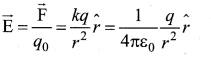Here r^ is the unit vector pointing from q to the point of interest P. The electric field is a vector quantity and its SI unit is Newton per Coulomb (NC-1).

Important aspects of the Electric field:
(i) If the charge q is positive then the electric field points away from the source charge and if q is negative, the electric field points towards the source charge q.

(ii) If the electric field at a point P is E⃗ , then the force experienced by the test charge qo placed at the point P is F⃗  = q0 E⃗ . This is Coulomb’s law in terms of the electric field. This is shown below Figure.

(iii) The equation implies that the electric field is independent of the test charge q0 and it depends only on the source charge q.

(iv) Since the electric field is a vector quantity, at every point in space, this field has a unique direction and magnitude as shown in Figures (a) and (b). From the equation, we can infer that as distance increases, the electric field decreases in magnitude. Note that in Figures (a) and (b) the length of the electric field vector is shown for three different points. The strength or magnitude of the electric field at point P is stronger than at the point Q and R because the point P is closer to the source charge.

(v) In the definition of the electric field, it is assumed that the test charge q0 is taken sufficiently small, so that bringing this test charge will not move the source charge. In other words, the test charge is made sufficiently small such that it will not modify the electric field of the source charge.

(vi) The expression is valid only for point charges. For continuous and finite-size charge distributions, integration techniques must be used. However, this expression can be used as an approximation for a finite-sized charge if the test point is very far away from the finite-sized source charge.

(vii) There are two kinds of electric field: uniform (constant) electric field and non-uniform electric field. A Uniform electric field will have the same direction and constant magnitude at all points in space. The non-uniform electric field will have different directions or different magnitudes or both at different points in space. The electric field created by a point charge is basically a non-uniform electric field. This non-uniformity arises, both in direction and magnitude, with the direction being radially outward (or inward), and the magnitude changes as distance increases.

Question 4.
How do we determine the electric field due to a continuous charge distribution? Explain. Electric field due to continuous charge distribution.
The electric charge is quantized microscopically. The expressions of Coulomb’s Law, superposition principle force and electric field are applicable to only point charges. While dealing with the electric field due to a charged sphere or a charged wire etc., it is very difficult to look at individual charges in these charged bodies. Therefore, it is assumed that charge is distributed continuously on the charged bodies, and the discrete nature of charges is not considered here. The electric field due to such continuous charge distributions is found by invoking the method of calculus.

Consider the following charged object of irregular shape. The entire charged object is divided into a large number of charge elements ∆q1, ∆q2, ∆q3 ……..∆qn,…… and each charge element Δq is taken as a point charge.
The electric field at a point P due to a charged object is approximately given by the sum of the fields at P due to all such charge elements
Here ∆ qi is the ith charge element, rip is the distance of the point P frome the ith charge element, rip is the unit vector from ith charge element to the pont P.
However the equation is only an approximation. To incorporate the continuous distribution of charge, we take the limit ∆q → 0(= dq). In this limit, the summation in the equation becomes an integration and takes the following form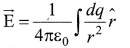Here r is the distance of the point P from the infinitesimal charge dq and r^ is the unit vector from dq to point P. Even though the electric field for a continuous charge distribution is difficult to evaluate, the force experienced by some test charge q in this electric field is still given by F⃗  = qE⃗ .

(a) Line charge distribution: If the charge Q is uniformly distributed along the wire of length L, then linear charge density (charge per unit length) is λ = QL. Its unit is colomb per meter (Cm-1). The charge present in the infinitestimal length dl is dq = λdl.

The electric field due to the line of total charge Q is given by

(b) Surface charge distribution: If the charge Q is uniformly distributed on a surface of area A, then surface charge density (charge per unit area) is σ = QA. Its unit is coulomb per square meter (C m-2). The charge present in the infinitesimal area dA is dq = σdA. The electric field due to a of total charge Q is given by

(c) Volume charge distribution: If the charge Q is uniformly distributed in a volume V, then volume charge density (charge per unit volume) is given by ρ = QV. Its unit is coulomb per cubic meter (Cm-3) The charge present in the infinitesimal volume element dV is dq = ρdV. The electric field due to a volume of total charge Q is given byQuestion 5.
Calculate the electric field due to a dipole on its axial line and the equatorial plane.
Case (I) :
Electric field due to an electric dipole at points on the axial line. Consider an electric dipole placed on the x-ax is as shown in the figure. A point C is located at a distance of r from the midpoint O of the dipole along the axial line. Axial line

The electric field at a point C due to +q is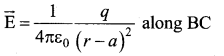Since the electric dipole moment vector P⃗  is from -q to +q and is directed along BC, the above equation is rewritten as

where p^ is the electric dipole moment unit vector from -q to +q. The electric field at a point C due to -q isSince +q is located closer to the point C than -q, E⃗  _. E⃗  + us stronger than E⃗ . Therefore, the length of the E + vector is drawn large than that of E⃗  _vector.
The total electric field at point C is calculated using the superposition principle of the electric field.

Note that the total electric field is along E⃗ , since +q is closer to C than -q.

The direction of E⃗ totis shown in Figure
If the point C is very far away from the dipole then (r >> a). Under this limit the term(r2 – a2)2 ≈ r4 Substituting this into equation, we get

If point C is chosen on the left side of the dipole, the total electric field is still in the

Case (II) :
Electric field due to an electric dipole at a point on the equatorial plane
Consider a point C at a distance r from the midpoint O of the dipole on the equatorial plane as shown in Figure. Since point C is equidistant from +q and -q, the magnitude of the electric fields of +q and -q are the same. The direction of E+ is along with BC and the direction of E is along with CA. E+ and E_ are resolved into two components; one component parallel to the dipole axis and the other perpendicular to it.

The perpendicular components ∣∣E⃗ +∣∣ sin θ and ∣∣E⃗ −∣∣ sin θ are oppositely directed and cancel each other. The magnitude of the total electric field at point C is the sum of the paralle component of E⃗ + and E⃗  and its direction is along −p^.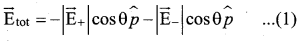The magnitudes E⃗ + and E⃗  are the same and are given by

By substituting equation (1) into equation (2), we get

At very large distances (r >> a), the equation becomes
E⃗ tot 14πε0 pr3 (r >>) …… (4)

Question 6.
Derive an expression for the torque experienced by a dipole due to a uniform electric field.
Torque experienced by an electric dipole in the uniform electric field:
Consider an electric dipole of dipole moment p⃗  placed in a uniform electric field E whose field lines are equally spaced and point in the same direction. The charge +q will experience a force qE⃗  in the direction of the field and charge -q will experience a force -qE⃗  in a direction opposite to the field.

Since the external field E⃗  is uniform, the total force acting on the dipole is zero. These two forces acting at different points will constitute a couple and the dipole experience a torque. This torque tends to rotate the dipole. (Note that electric field lines of a uniform field are equally spaced and point in the same direction). The total torque on the dipole about the point O
τ⃗  = OA−→− × (-qE⃗ ) + OB−→− × qE⃗
Using the right-hand corkscrew rule, it is found that total torque is perpendicular to the plane of the paper and is directed into it.

The magnitude of the total torque
τ⃗  = |OA−→−|(-qE⃗ ) sin θ + |OB−→−| |qE→| sin θ
where θ is the angle made by P⃗  with E⃗ . Since p = 2aq, the torque is written in terms of the vector product as
τ⃗  = p⃗  x E⃗
The magnitude of this torque is τ = pE sin θ and is maximum Torque on dipole
when θ =90°.
This torque tends to rotate the dipole and align it with the electric field E⃗ . Once E⃗  is aligned with E⃗ , the total torque on the dipole becomes zero.

Question 7.
Derive an expression for electrostatic potential due to a point charge.
Electric potential due to a point charge:
Consider a positive charge q kept fixed at the origin. Let P be a point at distance r from the charge q.
The electric potential at point P is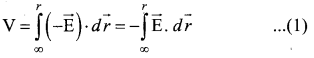Electric field due to positive point charge q is

The infinitesimal displacement vector, dr⃗  = drr^ and using r^ . r^ = 1, we have

After the integration,Hence the electric potential due to a point charge q at a distance r is
V = 14πε0 qr …… (2)
Important points (If asked in the exam)
(i) If the source charge q is positive, V > 0. If q is negative, then V is negative and equal to
V = 14πε0 qr
(ii) The description of the motion of objects using the concept of potential or potential energy is simpler than using the concept of field.
(iii) From expression (2), it is clear that the potential due to positive charge decreases as the distance increases, but for a negative charge, the potential increases as the distance are increased. At infinity (r = ∞) electrostatic potential is zero (V = 0).
(iv) The electric potential at a point P due to a collection of charges q1,q2,q3… qn is equal to the sum of the electric potentials due to individual charges.

Where r1, r2,r3,…..rn are the distances of q1,q2,q3… qn
respectively from P

Question 8.
Derive an expression for electrostatic potential due to an electric dipole.
Electrostatic potential at a point due to an electric dipole:
Consider two equal and opposite charges separated by a small distance 2a. The point P is located at a distance r from the midpoint of the dipole. Let 0 be the angle between the line OP and dipole axis AB.
Let r1 be the distance of point P from +q and r1 be the distance of point P from -q.
Potential at P due to charge +q = 14πε0 qr1
Potential at P due to charge -q = 14πε0 qr2
Total Potential at the point P,
V = 14πε0q (1r1−1r2) ….. (1)
Suppose if the point P is far away from the dipole, such that r >> a, then equation can be expressed in terms of r. By the cosine law for triangle BOP,

r21 = r2 + a2 – 2ra cos θ = r2 (1+a2r2−2arcosθ)
Since the point P is very far from dipole, then r >> a. As a result the term a2r2 is very small and can be neglected. Therefore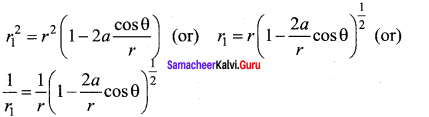since ar << 1, we can use binominal theorem and retain the terms up to first order
1r1 = (1+arcosθ) ……. (2)
Similarly applying the cosine law for triangle AOP,
r22 = r2 + a2 – 2ra cos (180 – θ)
Since cos (180 – θ) = cos θ we get
r22 = r2 + a2 + 2ra cos θ
Neglecting the term a2r2 (because r >> a)
r22 = r2 (1+2acosθr) (or) r2 = r (1+2acosθr)12
Using Binomial theorem, we get
1r2 = 1r (1−acosθr)
Substituting equations (3) and (2) in equation (1)But the electric dipole moment p = 2qa and we get,
V = 14πε0 (pcosθr2)
Now we can write p cos θ = P⃗ , r^ where r^ is the unit vector from the point O to point P. Hence the electric potential at a point P due to an electric dipole is given by
V = 14πε0 p⃗ ⋅r^r2 (r >> a) ….. (4)
Equation (4) is valid for distances very large compared to the size of the dipole. But for a point dipole, the equation (4) is valid for any distance.
Special cases:

since ar << 1, we can use binominal theorem and retain the terms up to first order
1r1 = (1+arcosθ) ……. (2)
Similarly applying the cosine law for triangle AOP,
r22 = r2 + a2 – 2ra cos (180 – θ)
Since cos (180 – θ) = cos θ we get
r22 = r2 + a2 + 2ra cos θ
Neglecting the term a2r2 (because r >> a)
r22 = r2 (1+2acosθr) (or) r2 = r (1+2acosθr)12
Using Binomial theorem, we get
1r2 = 1r (1−acosθr)
Substituting equations (3) and (2) in equation (1)

But the electric dipole moment p = 2qa and we get,
V = 14πε0 (pcosθr2)
Now we can write p cos θ = P⃗ , r^ where r^ is the unit vector from the point O to point P. Hence the electric potential at a point P due to an electric dipole is given by
V = 14πε0 p⃗ ⋅r^r2 (r >> a) ….. (4)
Equation (4) is valid for distances very large compared to the size of the dipole. But for a point dipole, the equation (4) is valid for any distance.
Special cases:

Case (I):
If the point P lies on the axial line of the dipole on the side of +q, then θ = 0. Then the electric potential becomes
V = 14πε0 pr2

Case (II):
If the point P lies on the axial line of the dipole on the side of -q, then θ = 180°, then
V = – 14πε0 pr2

Case (III):
If the point P lies on the equatorial line of the dipole, then θ = 90°. Hence, V = 0.

Question 9.
Obtain an expression for potential energy due to a collection of three-point charges which are separated by finite distances.
Electrostatic potential energy for a collection of point charges:
The electric potential at a point at a distance r from point charge ql is given by
V = 14πε0 q1r …… (1)
This potential V is the work done to bring a unit positive charge from infinity to the point. Now if the charge q2 is brought from infinity to that point at a distance r from qp the work done is the product of q2 and the electric potential at that point. Thus we have W = q2V …… (2)
This work done is stored as the electrostatic potential energy U of a system of charges q1 and q2 separated by a distance r. Thus we have
U = q2 V = 14πε0 q1q2r …… (3)
The electrostatic potential energy depends only on the distance between the two point charges. In fact, the expression (3) is derived by assuming that q1 is fixed and q2 is brought from infinity. The equation (3) holds true when q2 is fixed and q1 is brought from infinity or both q2and q2 are simultaneously brought from infinity to a distance r between them.
Three charges are arranged in the following configuration as shown in Figure.

To calculate the total electrostatic potential energy, we use the following procedure. We bring all the charges one by one and arrange them according to the configuration.
(i) Bringing a charge q1 from infinity to point A requires no work, because there are no other charges already present in the vicinity of charge q1

(ii) To bring the second charge q2 to point B, work must be done against the electric field created by the charge q1 So the work done on the charge q1 is W = q2V1B. Here V1B is the electrostatic potential due to the charge q1 at point B.
U = 14πε0 q1q2r12 ….. (4)
Note that the expression is the same when q2 is brought first and then q1 later.

(iii) Similarly to bring the charge q3 to point C, work has to be done against the total electric field due to both charges q1 and q2. So the work done to bring the charge q3 is = q3 (V1C + V2C). Here V1C is the electrostatic potential due to charge q1 at point C and V2C is the electrostatic potential due to charge q2 at point C. The electrostatic potential is

(iv) Adding equations (4) and (5), the total electrostatic potential energy for the system of three charges q1,q2, and q3 is

Note that this stored potential energy U is equal to the total external work done to assemble the three charges at the given locations. The expression (6) is the same if the charges are brought to their positions in any other order. Since the Coulomb force is a conservative force, the electrostatic potential energy is independent of the manner in which the configuration of charges is arrived at.

Question 10.
Derive an expression for the electrostatic potential energy of the dipole in a uniform electric field.
The electrostatic potential energy of a dipole in a uniform electric field:
Consider a dipole placed a torque when kept in an uniform electric field E⃗ . A dipole experiences a torque when kept in an uniform electric field E⃗ . This torque rotates the dipole to align it with the direction of the electric field. To rotate the dipole (at constant angular velocity) from its initial angle θ’ to another angle θ against the torque exerted by the electric field, an equal and opposite external torque must be applied on the dipole.

The work done by the external torque to rotate the dipole from angle θ’ to θ at constant angular velocity is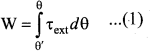Since τext is equal and opposite to τE = P⃗  x E⃗ , we have
∣∣r→ext∣∣ = ∣∣r→E∣∣= |P→×E→| …. (2)
Substituting equation (2) in equation (1) We get,

This work done is equal to the potential energy difference between the angular positions θ and θ’.
U(θ) – (Uθ’) = AU = -pE cos θ +PE cos θ’.
If the initial angle is = θ’ = 90° and is taken as reference point, then U(θ’) + pE cos θ’ = θ.
The potential energy stored in the system of dipole kept in the uniform electric field is given by El = -pE cos θ = –P⃗  . E⃗  ….. (3)
In addition to p and E, the potential energy also depends on the orientation θ of the electric dipole with respect to the external electric field.
The potential energy is maximum when the dipole is aligned anti-parallel (θ = π) to the external electric field and minimum when the dipole is aligned parallel (θ = 0) to the external electric field.

Question 11.
Obtain Gauss law from Coulomb’s law.
Gauss law: Gauss’s law states that if a charge Q is enclosed by an arbitrary closed surface, then the total electric flux ΦE through the closed surface is
ΦE = ∮E⃗  .d A⃗  = Qendε0
A positive point charge Q is surrounded by an imaginary sphere of radius r as shown in the figure. We can calculate the total electric flux through the closed surface of the sphere using the equation.

ΦE = ∮E⃗  .d A⃗  = ∮EdA cos θ …… (1)
The electric field of the point charge is directed radially outward at all points on the surface of the sphere. Therefore, the direction of the area element d A⃗  is along the electric field E⃗  and θ = 0°.
ΦE = ∮EdA since cos 0° = 1 ….. (2)
E is uniform on the surface of the sphere,
ΦE = ∮EdA ….. (3)
Substituting for
∮dA = 4π2 and E = 14πε0 Q in equation 3, we get
ΦE = 14πε0 qr2 × 4π2 = 4π 14πε0 = qε0 ……. (4)
The equation (4) is called as Gauss’s law. The remarkable point about this result is that the equation (4) is equally true for any arbitrary shaped surface which encloses the charge Q.

Question 12.
Obtain the expression for electric field due to an infinitely long charged wire.
Electric field due to an infinitely long charged wire:
Consider an infinitely long straight wire having uniform linear charge density λ. Let P be a point located at a perpendicular distance r from the wire. The electric field at the point P can be found using Gauss law. We choose two small charge elements A1 and A1 on the wire which are at equal distances from the point P.

The resultant electric field due to these two charge elements points radially away from the charged wire and the magnitude of electric field is same at all points on the circle of radius r. From this property, we can infer that the charged wire possesses a cylindrical symmetry.

Let us choose a cylindrical Gaussian surface of radius r and length L. The total electric flux in this closed surface is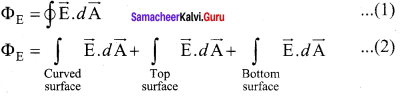It is seen that for the curved surface, E⃗  is parallel to A⃗  and E⃗ .d A⃗  = EdA. For the top and bottom surface, E⃗  is perpendicular to A⃗  and E⃗ .dA⃗  = 0
Substituting these values in equation (2) and applying Gauss law

Since the magnitude of the electric field for the entire curved surface is constant, E is taken out of the integration, and Qencl is given by Qencl = λL.

Here,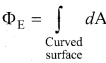dA = total area of the curved surface = 2πrL. Substituting this in
equation (4), We get

The electric field due to the infinite charged wire depends on 1r rather than 1r2 for a point charge.
Equation (6) indicates that the electric field is always along the perpendicular direction (r^ ) to wire. In fact, if λ > 0 then E points perpendicular outward (r^ ) from the wire and if λ < 0, then E points perpendicular inward (- r^ ).

Question 13.
Obtain the expression for the electric field due to a charged infinite plane sheet.
Electric field due to the charged infinite plane sheet: Consider an infinite plane sheet of charges with uniform surface charge density o. Let P be a point at a distance of r from the sheet. Since the plane is infinitely large, the electric field should be the same at all points equidistant from the plane and radially directed at all points. A cylindrical shaped Gaussian surface of length 2r and area A of the flat surfaces is chosen such that the infinite plane sheet passes perpendicularly through the middle part of the Gaussian surface.

Applying Gauss law for this cylindrical surface,

The electric field is perpendicular to the are element at all points on the curved surface and is parallel to the surface areas at P and P’. Then,

Since the magnitude of the electric field at these two equal surfaces is uniform, E is taken out of the integration, and Qencl is given by Qencl = σA, we get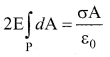The total area of surface either at P or P’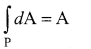Hence 2EA = σAε0 or E = σ2ε0 …… (3)
In vector from, E = σ2ε0 n^ ….. (4)
Hence n^ is the outward unit vector normal to the plane. Note that the electric field due to an infinite plane sheet of the charge depends on the surface charge density and is independent of the distance r.

The electric field will be the same at any point farther away from the charged plane. Equation (4) implies that if o > 0 the electric field at any point P is outward perpendicular n to the plane and if σ < 0 the electric field points inward perpendicularly (n^ ) to the plane. For a finite charged plane sheet, equation (4) is approximately true only in the middle region of the plane and at points far away from both ends.

Question 14.
Obtain the expression for the electric field due to a uniformly charged spherical shell.
Electric field due to a uniformly charged spherical shell:
Consider a uniformly charged spherical shell of radius R and total charge Q. The electric field at points outside and inside the sphere is found using Gauss law.

Case (a):
At a point outside the shell (r > R): Let us choose a point P outside the shell at a distance r from the center as shown in figure (a). The charge is uniformly distributed on the surface of the sphere (spherical symmetry). Hence the electric field must point radially outward if Q > 0 and point radially inward if Q < 0. So we choose a spherical Gaussian surface of radius r and the total charge enclosed by this Gaussian surface is Q. Applying Gauss law,
∮E⃗ .dA⃗  = Qε0 …….(1)
The electric field E⃗  and dA⃗  point in the same direction (outward normal) at all the points on the Gaussian surface. The magnitude of E⃗  is also the same at all points due to the spherical symmetry of the charge distribution.
ButdA = total area of Gaussian surface = 4πr2. Substituting this value in equation (2).

The electric field is radially outward if Q > 0 and radially inward if Q < 0. From equation (3), we infer that the electric field at a point outside the shell will be same as if the entire charge Q is concentrated at the center of the spherical shell. (A similar result is observed in gravitation, for gravitational force due to a spherical shell with mass M)

Case (b):
At a point on the surface of the spherical shell (r = R): The electrical field at points on the spherical shell (r = R) is given by
E⃗  = Q4πε0R2 r^ …… (4)

Case (c):
At a point inside the spherical shell (r < R): Consider a point P inside the shell at a distance r from the center. A Gaussian sphere of radius r is constructed as shown in the figure (b). Applying Gauss law

Since Gaussian surface encloses no charge, So Q = 0. The equation (5) becomes E = 0 (r < R) …(6)
The electric field due to the uniformly charged spherical shell is zero at all points inside the shell.

Question 15.
Discuss the various properties of conductors in electrostatic equilibrium.
Properties of conductors in electrostatic equilibrium:
(i) The electric field is zero everywhere inside the conductor. This is true regardless of whether the conductor is solid or hollow. This is an experimental fact. Suppose the electric field is not zero inside the metal, then there will be a force on the mobile charge carriers due to this electric field.

As a result, there will be a net motion of the mobile charges, which contradicts the conductors being in electrostatic equilibrium. Thus the electric field is zero everywhere inside – the conductor. We can also understand this fact by applying an external uniform electric field on the conductor.

Before applying the external electric field, the free electrons in the conductor are uniformly distributed in the conductor. When an electric field is applied, the free electrons accelerate to the left causing the left plate to be negatively charged and the right plate to be positively charged.

Due to this realignment of free electrons, there will be an internal electric field created inside the conductor which increases until it nullifies the external electric field. Once the external electric field is nullified the conductor is said to be in electrostatic equilibrium. The time taken by a conductor to reach electrostatic equilibrium is in the order of 10-6s, which can be taken as almost instantaneous.

(ii) There is no net charge inside the conductors. The charges must reside only on the surface of the conductors. We can prove this property using Gauss law. Consider an arbitrarily shaped conductor. A Gaussian surface is drawn inside the conductor such that it is very close to the surface of the conductor.

Since the electric field is zero everywhere inside the conductor, the net electric flux is also zero over this Gaussian surface. From Gauss’s law, this implies that there is no net charge inside the conductor. Even if some charge is introduced inside the conductor, it immediately reaches the surface of the conductor.

(iii) The electric field outside the conductor is perpendicular to the surface of the conductor and has a magnitude of σε0 where a is the surface charge density at that point. If the electric field has components parallel to the surface of the conductor, then free electrons on the surface of the conductor would experience acceleration. This means that the conductor is not in equilibrium. Therefore at electrostatic equilibrium, the electric field must be perpendicular to the surface of the conductor.

We now prove that the electric field has magnitude σε0 just outside the conductor’s surface. Consider a small cylindrical Gaussian surface. One half of this cylinder is embedded inside the conductor. Since electric field is normal to the surface of the conductor, the curved part of the cylinder has zero electric flux. Also inside the conductor, the electric field is zero. Hence the bottom flat part of the Gaussian surface has no electric flux. Therefore the top flat surface alone contributes to the electric flux. The electric field is parallel to the area vector and the total charge inside the surface is σA. By applying Gauss’s law,
EA = σAε0
In vector from, E⃗  = σε0 n^
Here n represents the unit vector outward normal to the surface of the conductor. Suppose σ < 0, then electric field points inward perpendicular to the surface.

(iv) The electrostatic potential has the same value on the surface and inside of the conductor. We know that the conductor has no parallel electric component on the surface which means that charges can be moved on the surface without doing any work. This is possible only if the electrostatic potential is constant at all points on the surface and there is no potential difference between any two points on the surface. Since the electric field is zero inside the conductor, the potential is the same as the surface of the conductor. Thus at electrostatic equilibrium, the conductor is always at equipotential.

Question 16.
Explain the process of electrostatic induction.
Whenever a charged rod is touched by another conductor, charges start to flow from the charged rod to the conductor. This type of charging without actual contact is called electrostatic induction:

(i) Consider an uncharged (neutral) conducting sphere at rest on an insulating stand. Suppose a negatively charged rod is brought near the conductor without touching it, as shown in figure (a). The negative charge of the rod repels the electrons in the conductor to the opposite side.

Various steps in electrostatic induction
As a result, positive charges are induced near the region of the charged rod while negative charges on the farther side. Before introducing the charged rod, the free electrons were distributed uniformly on the surface of the conductor and the net charge is zero. Once the charged rod is brought near the conductor, the distribution is no longer uniform with more electrons located on the farther side of the rod and positive charges are located closer to the rod. But the total charge is zero.

(ii) Now the conducting sphere is connected to the ground through a conducting wire. This is called grounding. Since the ground can always receive any amount of electrons, grounding removes the electron from the conducting sphere. Note that positive charges will not flow to the ground because they are attracted by the negative charges of the rod (figure (b)).

(iii) When the grounding wire is removed from the conductor, the positive charges remain near the charged rod (figure (c)).

(iv) Now the charged rod is taken away from the conductor. As soon as the charged rod is removed, the positive charge gets distributed uniformly on the surface of the conductor (figure (d)). By this process, the neutral conducting sphere becomes positively charged.

Question 17.
Explain dielectrics in detail and how an electric field is induced inside a dielectric.
Induced Electric field inside the dielectric:
When an external electric field is applied to a conductor, the charges are aligned in such a way that an internal electric field is created which cancels the external electric field. But in the case of a dielectric, which has no free electrons, the external electric field only realigns the charges so that an internal electric field is produced.

The magnitude of the internal electric field is smaller than that of the external electric field. Therefore the net electric field inside the dielectric is not zero but is parallel to an external electric field with a magnitude less than that of the external electric field. For example, let us consider a rectangular dielectric slab placed between two oppositely charged plates (capacitor) as shown in the figure.

The uniform electric field between the plates Induced electric field lines inside the dielectric acts as an external electric field E⃗ ext which polarizes the dielectric placed between plates. The positive charges are induced on one side surface and negative charges are induced on the other side of the surface But inside the dielectric, the net charge is zero even in a small volume. So the dielectric in the external field is equivalent to two oppositely charged sheets with the surface charge densities +σb and -σb. These charges are called bound charges. They are not free to move like free electrons in conductors. This is shown in the figure.

(a) Balloon sticks to the wall
(b) Polarisation of the wall due to the electric field created by the balloon
For example, the charged balloon after rubbing sticks onto a wall. The reason is that the negatively charged balloon is brought near the wall, it polarizes opposite charges on the surface of the wall, which attracts the balloon.

Question 18.
Obtain the expression for capacitance for a parallel plate capacitor.
The capacitance of a parallel plate capacitor:
Consider a capacitor with two parallel plates each of cross-sectional area A and separated by a distance d. The electric field between two infinite parallel plates is uniform and is given by E = σε0 where σ is the surface charge density on the plates σ = QA .If the separation distance d is very much smaller than the size of the plate (d2 << A), then the above result is used even for finite-sized
parallel plate capacitor.

The capacitance of a parallel plate capacitor
The electric field between the plates is
E = QAε0 ….. (1)
Since the electric field is uniform, the electric potential between the plates having separation d is given by
V = Ed = QdAε0 ….. (2)
Therefore the capacitance of the capacitor is given by
C = QV = Q(QdAε0) = ε0Ad ….. (3)
From equation (3), it is evident that capacitance is directly proportional to the area of cross-section and is inversely proportional to the distance between the plates. This can be understood from the following.

• If the area of cross-section of the capacitor plates is increased, more charges can be distributed for the same potential difference. As a result, the capacitance is increased.
• If the distance d between the two plates is reduced, the potential difference between the plates (V = Ed) decreases with the E constant.

Question 19.
Obtain the expression for energy stored in the parallel plate capacitor.
Energy stored in the capacitor:
Capacitor not only stores the charge but also it stores energy. When a battery is connected to the capacitor, electrons of total charge -Q are transferred from one plate to the other plate. To transfer the charge, work is done by the battery. This work done is stored as electrostatic potential energy in the capacitor. To transfer an infinitesimal charge dQ for a potential difference V, the work done is given by
dW = VdQ ….. (1)
Where V = QC
The total work done to charge a capacitor is

This work done is stored as electrostatic potential energy (UE) in the capacitor.
UE = Q22C = 12 CV2 (∴ Q = CV) ….. (3)
where Q = CV is used. This stored energy is thus directly proportional to the capacitance of the capacitor and the square of the voltage between the plates of the capacitor. But where is this energy stored in the capacitor? To understand this question, the equation (3) is rewritten as follows using the results
C = ε0Ad and V = Ed
where Ad = volume of the space between the capacitor plates. The energy stored per unit volume of space is defined as energy density Volume¯¯¯¯¯¯¯¯¯¯¯¯¯¯¯¯¯. Frome equation (4) we get
uE = 12 ε0E2
From equation (5), we infer that the energy is stored in the electric field existing between the plates of the capacitor. Once the capacitor is allowed to discharge, the energy is retrieved.

Question 20.
Explain in detail the effect of a dielectric placed in a parallel plate capacitor.
(i) When the capacitor is disconnected from the battery:
Consider a capacitor with two parallel plates each of cross-sectional area A and are separated by a distance d. The capacitor is charged by a battery of voltage V0 and the charge stored is Q0. The capacitance of the capacitor without the dielectric is
C0 = Q0V0 ….. (1)
The battery is then disconnected from the capacitor and the dielectric is inserted between the plates. The introduction of dielectric between the plates will decrease the electric field. Experimentally it is found that the modified electric field is given by

(a) Capacitor is charged with a battery
(b) Dielectric is inserted after the battery is disconnected
E = E0εr …… (2)
Here E0 is the electric field inside the capacitors when there is no dielectric and εr is the relative permeability of the dielectric or simply known as the dielectric constant. Since εr > 1, the electric field E < E0. As a result, the electrostatic potential difference between the plates (V = Ed) is also reduced. But at the same time, the charge Q0 will remain constant once the battery is disconnected. Hence the new potential difference is
V = Ed = E0εrd = V0εr ….. (3)
We know that capacitance is inversely proportional to the potential difference. Therefore as V decreases, C increases. Thus new capacitance in the presence of a dielectric is
C = Q0V = εr Q0V0 = εr C0 …… (4)
Since εr > 1, we have C > C0. Thus insertion of the dielectric constant εr increases the capacitance. Using equation,
where ε = εrε0 is the permittivity of the dielectric medium. The energy stored in the capacitor before the insertion of a dielectric is given by U0 = 12 Q20C0 ….. (6)
After the dielectric is inserted, the charge Q0 remains constant but the capacitance is increased. As a result, the stored energy is decreased.

Since εr> 1 we get U < U0. There is a decrease in energy because, when the dielectric is inserted, the capacitor spends some energy in pulling the dielectric inside.

(ii) When the battery remains connected to the capacitor: Let us now consider what happens when the battery of voltage V0 remains connected to the capacitor when the dielectric is inserted into the capacitor.
The potential difference V0 across the plates remains constant. But it is found experimentally (first shown by Faraday) that when the dielectric is inserted, the charge stored in the capacitor is increased by a factor εr.

(a) Capacitor is charged through a battery
(b) Dielectric is inserted when the battery is connected.
Q = εrQ0 ….. (1)
Due to this increased charge, the capacitance is also increased. The new capacitance is
C = Q0V = εr Q0V0 = εr C0 …… (2)
However, the reason for the increase in capacitance in this case when the battery remains connected is different from the case when the battery is disconnected before introducing the dielectric.
U0 = 12 C0 V20 ….. (4)
Note that here we have not used the expression
U0 = 12V20C0
because here, both charge and capacitance are changed, whereas in equation 4, V0 remains constant. After the dielectric is inserted, the capacitance is increased; hence the stored energy is also increased.
U = 12 CV20 = 12 εr CV20 = εr U0
Since er > 1 we have U > U0
It may be noted here that since voltage between the capacitor V0 is constant, the electric field between the plates also remains constant.

Question 21.
Derive the expression for resultant capacitance, when capacitors are connected in series and in parallel.
capacitors in series and parallel:
(i) Capacitors in series:
Consider three capacitors of capacitance C1, C2 and C3 connected in series with a battery of voltage V as shown in figure (a).
As soon as the battery is connected to the capacitors in series, the electrons of charge -Q are transferred from the negative terminal to the right plate of C3which pushes the electrons of the same amount -Q from left plate of C3 to the right plate of C2 due to electrostatic induction. Similarly, the left plate of C2 pushes the charges of Q to the right plate of which induces the positive charge +Q on the left plate of C1 At the same time, electrons of charge -Q are transferred from the left plate of C1 to the positive terminal of the battery.

By these processes, each capacitor stores the same amount of charge Q. The capacitances of the capacitors are in general different so that the voltage across each capacitor is also different and are denoted as V1, V2 and V3 respectively.
The total voltage across each capacitor must be equal to the voltage of the battery.
V = V1 + V2 + V3 ….. (1)
Since Q = CV, we have V = QC1 + QC2 + QC3
Q = (1C1+1C2+1C3) ….. (2)
If three capacitors in series are considered to form an equivalent single capacitor Cs shown in figure (b), then we have V = QCs
Substituting this expression into equation (2) we get
V = QCs = Q(1C1+1C2+1C3)
1Cs = 1C1 + 1C2 + 1C3 ….. (3)
Thus, the inverse of the equivalent capacitance Cs of three capacitors connected in series is equal to the sum of the inverses of each capacitance. This equivalent capacitance Cs is always less than the smallest individual capacitance in the series.

(ii) Capacitance in parallel:
Consider three capacitors of capacitance C1,C2 and C3 connected in parallel with a battery of voltage V as shown in figure (a).

Since corresponding sides of the capacitors are connected to the same positive and negative terminals of the battery, the voltage across each capacitor is equal to the battery’s voltage. Since the capacitance of the capacitors is different, the charge stored in each capacitor is not the same. Let the charge stored in the three capacitors be Q1,Q2, and Q2 respectively. According to the law of conservation of total charge, the sum of these three charges is equal to the charge Q transferred by the battery,
Q = Q1 + Q2 + Q3 ….. (1)
Now, since Q = CV, we have
Q = C1V + C2 V + C3 V ….. (2)
If these three capacitors are considered to form a single capacitance CP which stores the total charge Q as shown in figure (b), then we can write Q = CPV. Substituting this in equation (2), we get
Cp V = CV + C2 V + C3 V
Cp = C1 + C2 + C3
Thus, the equivalent capacitance of capacitors connected in parallel is equal to the sum of the individual capacitance. The equivalent capacitance Cp in a parallel connection is always greater than the largest individual capacitance. In a parallel connection, it is equivalent as an area of each capacitance adds to give a more effective area such that total capacitance increases.

Class 12 physics formula pdf in hindi 2022 |

कक्षा 12 भौतिक विज्ञान महत्वपूर्ण फार्मूला पीडीएफ़ 2022

Mp Board Time Table 2022 class 12th |

एमपी बोर्ड कक्षा 12 मॉडल पेपर 2022 पीडीएफ़ डाउनलोड